13.5 Eddy currents  (Page 2/4)

 Page 2 / 4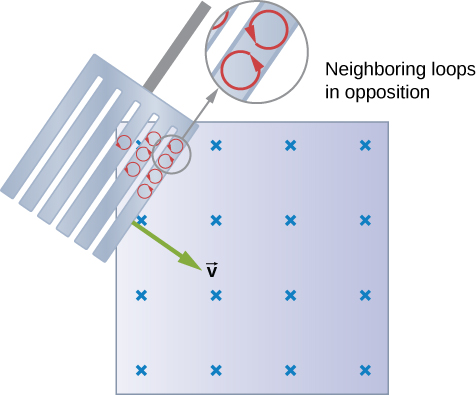Eddy currents induced in a slotted metal plate entering a magnetic field form small loops, and the forces on them tend to cancel, thereby making magnetic drag almost zero.

Applications of magnetic damping

One use of magnetic damping is found in sensitive laboratory balances. To have maximum sensitivity and accuracy, the balance must be as friction-free as possible. But if it is friction-free, then it will oscillate for a very long time. Magnetic damping is a simple and ideal solution. With magnetic damping    , drag is proportional to speed and becomes zero at zero velocity. Thus, the oscillations are quickly damped, after which the damping force disappears, allowing the balance to be very sensitive ( [link] ). In most balances, magnetic damping is accomplished with a conducting disc that rotates in a fixed field.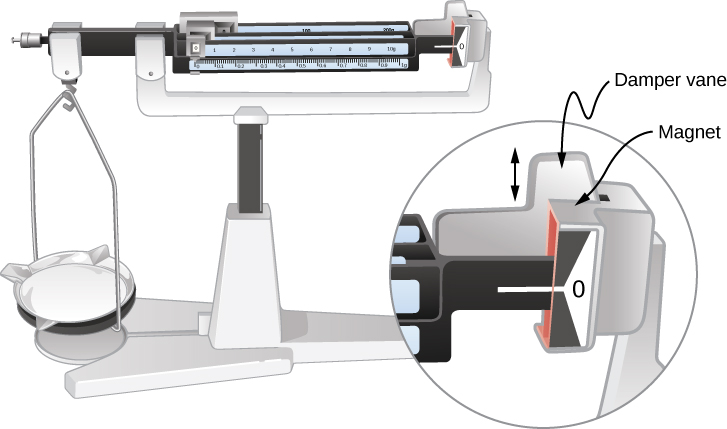Magnetic damping of this sensitive balance slows its oscillations. Since Faraday’s law of induction gives the greatest effect for the most rapid change, damping is greatest for large oscillations and goes to zero as the motion stops.

Since eddy currents and magnetic damping occur only in conductors, recycling centers can use magnets to separate metals from other materials. Trash is dumped in batches down a ramp, beneath which lies a powerful magnet. Conductors in the trash are slowed by magnetic damping while nonmetals in the trash move on, separating from the metals ( [link] ). This works for all metals, not just ferromagnetic ones. A magnet can separate out the ferromagnetic materials alone by acting on stationary trash.Metals can be separated from other trash by magnetic drag. Eddy currents and magnetic drag are created in the metals sent down this ramp by the powerful magnet beneath it. Nonmetals move on.

Other major applications of eddy currents appear in metal detectors and braking systems in trains and roller coasters. Portable metal detectors ( [link] ) consist of a primary coil carrying an alternating current and a secondary coil in which a current is induced. An eddy current is induced in a piece of metal close to the detector, causing a change in the induced current within the secondary coil. This can trigger some sort of signal, such as a shrill noise.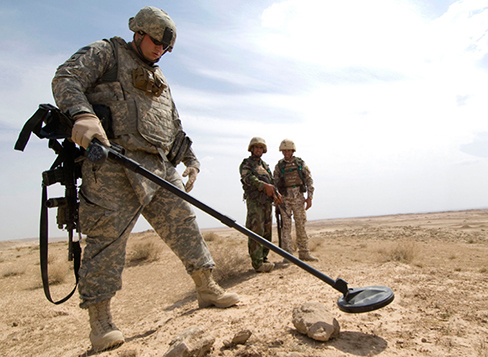A soldier in Iraq uses a metal detector to search for explosives and weapons. (credit: U.S. Army)

Braking using eddy currents is safer because factors such as rain do not affect the braking and the braking is smoother. However, eddy currents cannot bring the motion to a complete stop, since the braking force produced decreases as speed is reduced. Thus, speed can be reduced from say 20 m/s to 5 m/s, but another form of braking is needed to completely stop the vehicle. Generally, powerful rare-earth magnets such as neodymium magnets are used in roller coasters. [link] shows rows of magnets in such an application. The vehicle has metal fins (normally containing copper) that pass through the magnetic field, slowing the vehicle down in much the same way as with the pendulum bob shown in [link] .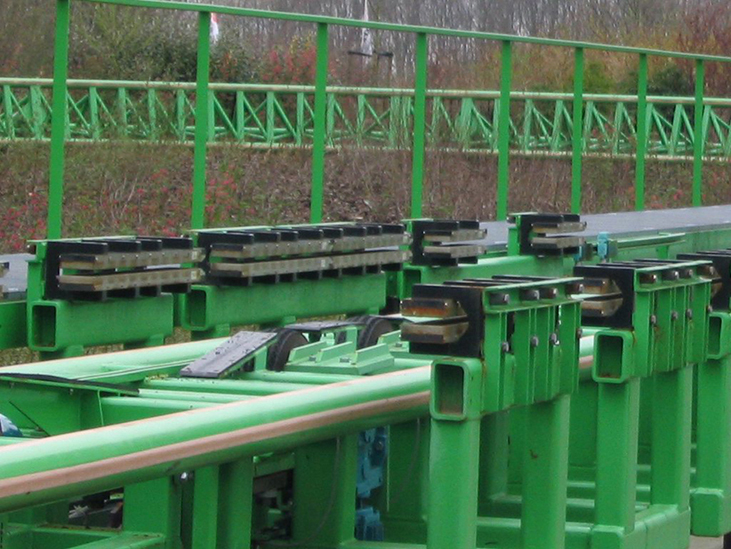The rows of rare-earth magnets (protruding horizontally) are used for magnetic braking in roller coasters. (credit: Stefan Scheer)

Induction cooktops have electromagnets under their surface. The magnetic field is varied rapidly, producing eddy currents in the base of the pot, causing the pot and its contents to increase in temperature. Induction cooktops have high efficiencies and good response times but the base of the pot needs to be conductors, such as iron or steel, for induction to work.

Summary

• Current loops induced in moving conductors are called eddy currents. They can create significant drag, called magnetic damping.
• Manipulation of eddy currents has resulted in applications such as metal detectors, braking in trains or roller coasters, and induction cooktops.

Conceptual questions

A conducting sheet lies in a plane perpendicular to a magnetic field $\stackrel{\to }{B}$ that is below the sheet. If $\stackrel{\to }{B}$ oscillates at a high frequency and the conductor is made of a material of low resistivity, the region above the sheet is effectively shielded from $\stackrel{\to }{B}$ . Explain why. Will the conductor shield this region from static magnetic fields?

The conducting sheet is shielded from the changing magnetic fields by creating an induced emf. This induced emf creates an induced magnetic field that opposes any changes in magnetic fields from the field underneath. Therefore, there is no net magnetic field in the region above this sheet. If the field were due to a static magnetic field, no induced emf will be created since you need a changing magnetic flux to induce an emf. Therefore, this static magnetic field will not be shielded.

Electromagnetic braking can be achieved by applying a strong magnetic field to a spinning metal disk attached to a shaft. (a) How can a magnetic field slow the spinning of a disk? (b) Would the brakes work if the disk was made of plastic instead of metal?

A coil is moved through a magnetic field as shown below. The field is uniform inside the rectangle and zero outside. What is the direction of the induced current and what is the direction of the magnetic force on the coil at each position shown?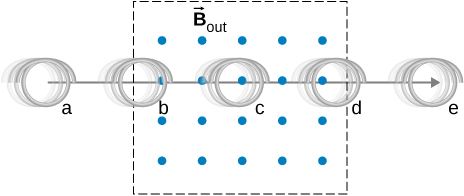a. zero induced current, zero force; b. clockwise induced current, force is to the left; c. zero induced current, zero force; d. counterclockwise induced current, force is to the left; e. zero induced current, zero force.

in the 2nd example, for chapter 8.2 on page 3/3, I don't understand where the value 48uC comes from, I just couldn't get that value in my calculator.
what is electromagnetic force. do electric and magnetic force happen differently
yes
yes
Pranay
why
Godson
how?
Godson
derived the electric potential due to disk of charge
how can we derived potential electric due to the disk
aron
how can you derived electric potential of a disk
aron
how can you derived electric potential due to disk
aron
where is response?
aron
what is difference between heat and temperature?
temperature is the measure of degree of hotness or coldness. on the other hand, heat is the form of energy, which causes temperature. So we can safely say, heat is the reason and temperature is its consequence.
SABYASACHI
how many liquid metals do we have
do we have gasses as metals
Jeffery
who knows should please tell us
yes...gallium & cesium
Idris
Hg is liquid. No metal gasses at standard temp and pressure
Shane
I don't ever understand any of this formulae
which formula
How to determine a temperature scale
what is the formula for absolute error
Nyro
define kelvin planck statement
using p-v diagram, explain what takes place during each four processes of a carnot cycle
Masia
using p-v diagram, explain what takes place during each four processes of a carnot cycle
Masia
state zeroths law of thermodynamics
The zeroth law of thermodynamics states that if twothermodynamic systems are each in thermal equilibrium with a third one, then they are in thermal equilibrium with each other
Seza
zeroth law of thermodynamic state that when a body A and B are in thermal equilibrium to body C ,a state is attained when body A,B and C are in thermal equilibrium.
akanbi
sorry guys I got no any idea on the law can someone help me please?
SNAHR
hi
Muhsin
The zeroth law of thermodynamics states that if two thermodynamic systems are each in thermal equilibrium with a third one, then they are in thermal equilibrium with each other. Accordingly, thermal equilibrium between systems is a transitive relation. Two systems are said to be in the relation of
Muhsin
I can help u Snahr as possible
Vinayaka
it simply states that, if two bodies are separately in thermal equilibrium with a third body, then those two bodies will also be in thermal equilibrium with each other
Vinayaka
What mass of steam of 100 degree celcius must be mixed with 150g of ice at 0 degree celcius, in a thermally insulated container, to produce liquid water at 50 degree celcius
sorry I dont know
Bamidele
thank you
Emmanuel
What is the pressure?
SHREESH
To convert 0°C ice to 0°c water. Q=M*s=150g*334J/g=50100 J.......... Now 0° water to 50° water... Q=M*s*dt=150g*4.186J/g*50= 31395 J....... Which adds upto 81495 J..... This is amount of heat the steam has to carry. 81495= M *s=M*2230J/g..therefore.....M=36.54g of steam
SHREESH
This is at 1 atm
SHREESH
If there is change in pressure u can refer to the steam table ....
SHREESH
instrument for measuring highest temperature of a body is?
Thermometer
Umar
how does beryllium decay occur
Photon?
Umar
state the first law of thermodynamics
Its state that "energy can neither be created nor destroyed but can be transformed from one form to another. "
Ayodamola
what about the other laws can anyone here help with it please
Sandy
The second law of thermodynamics states that the entropy of any isolated system always increases. The third law of thermodynamics states that the entropy of a system approaches a constant value as the temperature approaches absolute zero.
sahil
The first law is very simple to understand by its equation. The law states that "total energy in thermodynamic sytem is always constant" i.e d¶=du+dw where d¶=total heat du=internal energy dw=workdone... PLEASE REFER TO THE BOOKS FOR MORE UNDERSTANDING OF THE CONCEPT.
Elia
what is distance.?
what is physics?
Ali
Physics is a scientific phenomenon that deals with matter and its properties
Ayodamola
physics is the study of nature and science
John
Chater1to7
min
Physics is branch of science which deals with the study of matters in relation with energy.
Elia
What is differential form of Gauss's law?ByByByByBy